SAT  >  Test: Exponents and Radicals - 1

# Test: Exponents and Radicals - 1

Test Description

## 10 Questions MCQ Test Mathematics for SAT | Test: Exponents and Radicals - 1

Test: Exponents and Radicals - 1 for SAT 2023 is part of Mathematics for SAT preparation. The Test: Exponents and Radicals - 1 questions and answers have been prepared according to the SAT exam syllabus.The Test: Exponents and Radicals - 1 MCQs are made for SAT 2023 Exam. Find important definitions, questions, notes, meanings, examples, exercises, MCQs and online tests for Test: Exponents and Radicals - 1 below.
Solutions of Test: Exponents and Radicals - 1 questions in English are available as part of our Mathematics for SAT for SAT & Test: Exponents and Radicals - 1 solutions in Hindi for Mathematics for SAT course. Download more important topics, notes, lectures and mock test series for SAT Exam by signing up for free. Attempt Test: Exponents and Radicals - 1 | 10 questions in 15 minutes | Mock test for SAT preparation | Free important questions MCQ to study Mathematics for SAT for SAT Exam | Download free PDF with solutions
 1 Crore+ students have signed up on EduRev. Have you?
Test: Exponents and Radicals - 1 - Question 1

### If 3(x+y) = 81 and 81(x−y) = 3, then the value of x is

Detailed Solution for Test: Exponents and Radicals - 1 - Question 1

3x+y = 81
3x+y = 34
x + 4 = 4....(1)
81x-y = 3
(34)x-y = 3
4(x - y) = 3
34(x-y) = 1
x - y = 1/4....(2)
(1) + (2)
2x = 17/4
x = 17/8

Test: Exponents and Radicals - 1 - Question 2

###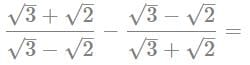Detailed Solution for Test: Exponents and Radicals - 1 - Question 2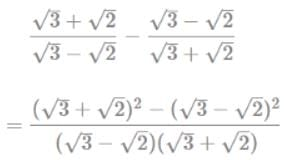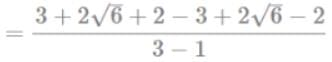4√6

Test: Exponents and Radicals - 1 - Question 3

### If 2x = ∛32, then x=

Detailed Solution for Test: Exponents and Radicals - 1 - Question 3

2x = ∛32
2x = (2)5/3
x = 5/3

Test: Exponents and Radicals - 1 - Question 4

Given that √5 = 2.236 and √3 = 1.732, then the value of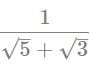is

Detailed Solution for Test: Exponents and Radicals - 1 - Question 4

√5 = 2.236
√3 = 1.732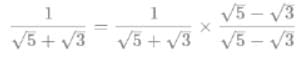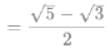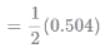0.252

Test: Exponents and Radicals - 1 - Question 5

If 10= 1/2 then 10−8x =

Detailed Solution for Test: Exponents and Radicals - 1 - Question 5

10x = 1/2
10-8x = (10x)−8
= (1/2)−8
= 28
= 256

Test: Exponents and Radicals - 1 - Question 6

The value of 51/4 × (125)0.25 is

Detailed Solution for Test: Exponents and Radicals - 1 - Question 6

51/4 × (125)0.25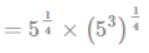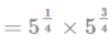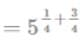= 5

Test: Exponents and Radicals - 1 - Question 7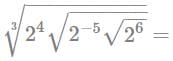Detailed Solution for Test: Exponents and Radicals - 1 - Question 7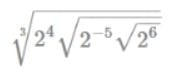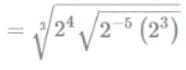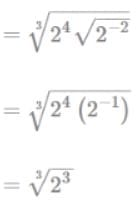= 2

Test: Exponents and Radicals - 1 - Question 8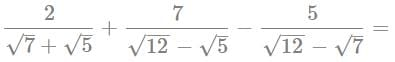Detailed Solution for Test: Exponents and Radicals - 1 - Question 8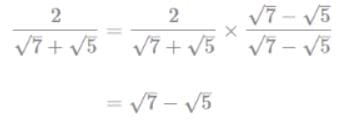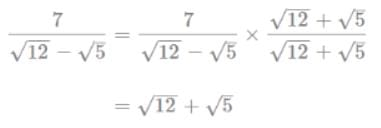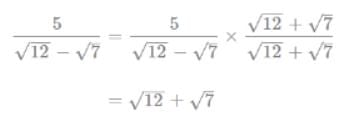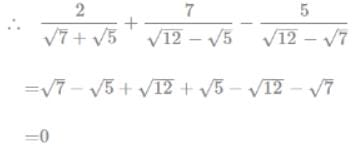Test: Exponents and Radicals - 1 - Question 9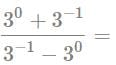Detailed Solution for Test: Exponents and Radicals - 1 - Question 9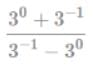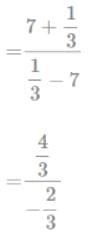= -2

Test: Exponents and Radicals - 1 - Question 10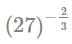lies between

Detailed Solution for Test: Exponents and Radicals - 1 - Question 10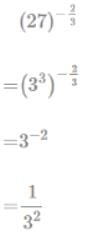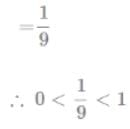## Mathematics for SAT

184 videos|111 docs|67 tests(Scan QR code)